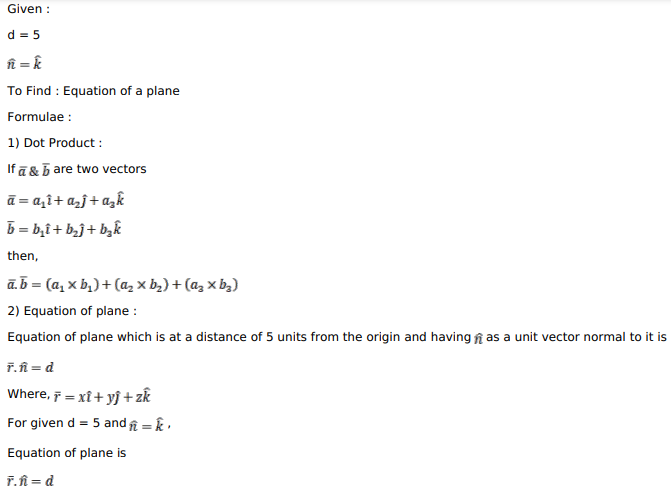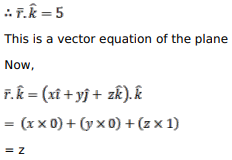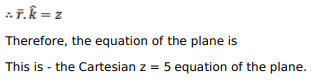# Find the vector and Cartesian equations of a plane which is at a distanceQuestion:

Find the vector and Cartesian equations of a plane which is at a distance of 5 units from the origin and which has $\hat{k}$ as the unit vector normal to it.

Solution: